# AP Physics 1 Question 317: Answer and Explanation

### Test Information

Question: 317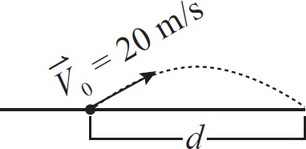5. A soccer ball on a level field is kicked at an angle of 30 degrees above the horizontal with an initial speed of 20 m/s, as drawn above. What is d, the distance from the spot of the kick to the spot where the ball lands? Ignore air resistance.

• A.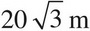• B. 40 m
• C.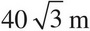• D. 80 m

Explanation:

A

First, split up v0 into horizontal and vertical components.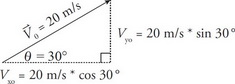vx0 = 20 m/s *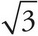/2 = 10m/s, vy0 = 20 m/s * 1/2 = 10 m/s. Then, make a column of vertical values. Take the positive direction to be up.

Vertical

y - y0 = 0 (since it starts and finishes on the ground)

vy0 = 10 m/s

vyf = don't care

ay = -10 m/s2

t = ?

Plugging these into Big Five #3 gives t = 0 s or 2 s, meaning there are two times at which the elevation of the ball is 0 m. (What is Big Five #3? Or, better yet, what are the 5 Big Five equations that we love so much? We cover them all in Chapter 4: Kinematics. Flip there now to learn more or come back to this page later for further clarification.) Zero seconds corresponds to the instant the ball leaves the ground; 2 seconds corresponds to the time the ball lands on the ground. (Alternatively, you could use Big Five #2 to calculate the time the ball spends traveling up, and then double it to get the total time in flight, as done in Examples 25 and 26 of Chapter 4.)

Finally, make your column of horizontal values.

Horizontal

x - x0 = ?

vx = 10m/s (remember, forward speed is constant for an ideal projectile)

t = 2 s (You just solved for this.)

Solve for the horizontal displacement by using the definition of velocity, x - x0 = vxt, or just d = vxt. This gives d = (10m/s) * 2 s = 20m/s.